# An example of the initial phases of software developmentConsidering the previously mentioned phases of software development, according to the example of tools for programming, it is clear that regardless of the choice of language processor first four stages are always the same. The differences are in the writing of program code for each language processors and thus further in the examples will not show the process of the fifth stage onwards.

The first four phases of the development of software (algorithm and flowchart) to convert between numeric systems.

1. phase
Develop conversion between numeric system according to the principle: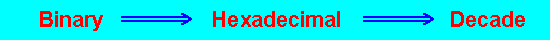2. phase
Conversion performed by the binary number up to 16 bits and convert the hexadecimal equivalent of the decade.

3. phase
a.) Allow entry digit binary number B into a matrix of 16 elements,
the value of the variable n with boundary { 0 < n < 15 }.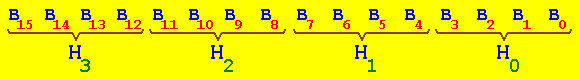b.) Compute elements of hexadecimal number H by the expression: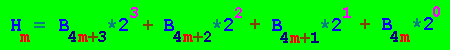Value of variable m will be within the limits { 0 < m < 3 }.
If Hm >9 let's then: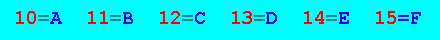The result is a hexadecimal number: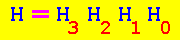c.) Decade calculate the value of D according to the formula: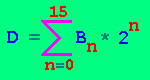4. phase
Production of block diagram (flowchart) that will be done for programming.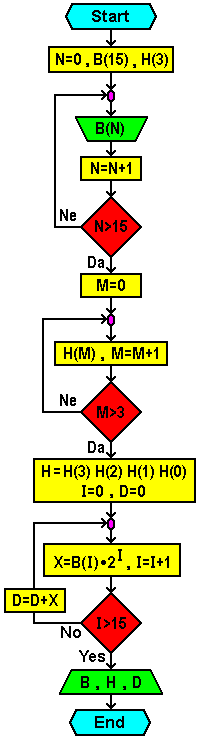Citing of this page: Radic, Drago. " IT - Informatics Alphabet " Split-Croatia. {Date of access}. . Copyright © by Drago Radic. All rights reserved. | Disclaimer